Warning

# Convolutional Neural Networks

In this lab, we will learn how to build and train Convolutional Neural Networks (CNN) using the MatConvNet framework. In particular, we will train several different CNNs for the recognition of handwritten digits (0-9) from 28×28 grayscale images.

We will build the network step-by-step demonstrating various techniques used nowadays as well as several caveats one should be aware of.

There are many resources available today for learning more about deep learning and CNNs in particular. You can find a few useful ones at the bottom of this page. However, hold on reading them all first. We will go slowly, adding one component after another and when you are finally set to do your own coding, you may want to revisit these links or find your own lectures, tutorials, videos, … We added links to relevant explanations into the text itself, so whenever you meet a new term, you can learn its meaning.

The output of this assignment will be a single file my_cnn.mat containing a trained CNN digit classifier and the scripts used for training packed together in a single .zip file. See the template code for a way how to save the network.

The challenge: The network will be automatically evaluated by the upload system and ranked in the online score board. The gained points from the assignment depend on the rank of your algorithm:

• 1st place: 16 points
• 2nd place: 14 points
• 3rd place: 12 points
• 4th place: 10 points
• every submission with performance worse than the baseline: 0 points
• 8 points otherwise

Deadline for the submission is Sun Jan 6 23:59. Then the points will be assigned. Every later submission is then for 6 points. You have to be better than the baseline to complete this lab.

## MatConvNet Instalation

There are several popular frameworks for training CNNs (Caffe, TensorFlow, Torch, Theano). Feel free to explore and try them after mastering this lab. For simplicity we will stay within the Matlab environment and will use the MatConvNet framework.

Download and unzip matconvnet-1.0-beta23.tar.gz. Start a Matlab session and type the following commands in order to go into the unzipped directory, compile MEX-files and set necessary paths.

>> cd matconvnet-1.0-beta23
>> run matlab/vl_compilenn
>> run matlab/vl_setupnn

For Windows, the compiled .mex files are here.

## Data description

We have prepared an image training dataset MAT-file (imdb.mat) with images and their corresponding labels from the MNIST dataset hosted on Yann Le Cun's website. It can be loaded using load imdb.mat command. It creates a structure imdb in Matlab with the following fields:

• imdb.images.data contains 28x28x1 dimensional images concatenated in a 4D array.
• imdb.images.labels contains corresponding labels (i.e. labels 1-10, which correspond to digits 0-9).
• imdb.images.data_mean contains mean which has been subtracted from the original images to make the training easier.

During our initial testing of the system everything seemed alright, until we discovered that at the test time our customer uses a low quality camera with a lot of noise. Thus, the handwriting style stays the same, but the images are heavily corrupted (see the images below). Since annotating these noisy images is costly, we were able to correctly label only a small fraction of them - only 1000 images.

Thus, you are given 60000 annotated images, where the first 59000 (1:59000) are the clean images from MNIST and the rest (59001:60000) come from the noisy camera. We recommend keeping the last 1000 images as a validation set and augment the clean MNIST images (add noise to them) in order to get enough relevant training data. We do not know the exact noise characteristics of the camera, but the provided 1000 validation samples should help you to estimate the noise type and its intensity (image noise), as well as validate your results before submitting them to the upload system (the network is evaluated only on noisy images for test purposes!).

Feel free to use also the clean MNIST images for training, but according to our initial tests, they are not sufficient to produce a good enough classifier and thus augmentation is necessary.

Examples of the clean MNIST data: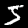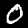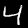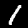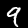Examples of the noisy data from the camera: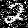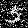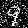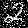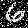## 1. Linear classifier, softmax regression, stochastic gradient descent

We start with a simple one layer fully-connected network to demonstrate the basic principles. First, in contrast to previous algorithms, neural networks extend to the multi-class case quite naturally. This is an extension of the logistic regression.

Lets denote the images as $\mathbf{x}\in\mathcal{R}^{28\times 28}$ and the labels as $y\in \{0,1\dots,9\}$. In the binary logistic regression the probability that an image $\mathbf{x}$ has label $y=1$ was modelled by $$P(y=1|\mathbf{x},w) = \frac{1}{1+\mathrm{exp}(\mathbf{w}^\top\mathrm{vec}(\mathbf{x}))}$$ The softmax regression generalises this expression to $K$ classes. The probability that an image $\mathbf{x}$ has a label $y=k$ is modelled as $$P(y=k|\mathbf{x},w) = \frac{\mathrm{exp}(\mathbf{w}_k^\top\mathrm{vec}(\mathbf{x}))}{\sum_{j=1}^{K}\mathrm{exp}(\mathbf{w}_j^\top\mathrm{vec}(\mathbf{x}))}$$ where $\mathbf{w}_k$ is the vector of weights of $k$-th output neuron.

As shown in the lecture slides, the go-to learning algorithm in neural networks is the back-propagation using gradient descent. If you study the formulas carefully, they contain a sum over all training examples. With increasing the training set size (which we want for difficult problems), this becomes prohibitive. Instead, one uses stochastic gradient descent (SGD).

The basic idea of the stochastic gradient descent is simple: Take a smaller portion of the data (called a batch) and use it to estimate the gradient. This way only an estimate of the gradient is obtained. Using it to update the network weights is thus not optimal, but generally guides the optimisation in the right direction.

• Prove that the softmax regression is a generalisation of the logistic regression, i.e. show that for a binary classification problem ($K$=2) the softmax model is equivalent to the logistic model. (Only when desperate, have a look at the solution)

The template contains a code for training a simple one layer network with a softmax regression on the output and trained using the stochastic gradient descent. MatConvNet stores the layers of a neural network in a structure array. For example a convolutional layer, which computes convolution of the input image (input_rows x input_cols x input_channels) with N convolutional kernels (N=output_channels) is initialised as follows:

>> net.layers{1} = struct('type', 'conv', ...
'weights', {{1e-2*randn(input_rows,input_cols,input_channels, output_channels,'single'), randn(1,output_channels,'single')}}, ...
'stride', 1, ...
'pad', 0) ;
The second randn in the weights initialisation is for the bias term ($y=wx+b$). In our case, we want to build a neural network with a single fully connected layer. This is implemented in MatConvNet as a CNN layer configured to act as a fully-connected layer with input of size 28x28x1 and with 10 outputs corresponding to probabilities $P(x|y=k)$.

In order to train a CNN, a loss function must be defined. MatConvNet implements the loss function as a special layer (e.g. see the softmax layer).

1. Train the network on the MNIST data.
>> [net, info] = cnn_train(net, imdb, @getSimpleNNBatch, 'batchSize', 1000, 'numEpochs', 100, 'expDir', 'expDir');
2. It is always a good idea to train another standard classifier (e.g. SVM) on the same data to get some baseline results. If the network does not perform better, something is wrong. Try to train an SVM classifier with the same data.

3. Add another fully-connected layer with 1000 hidden units and with sigmoid non-linearity. Do you get better results?.

4. Try to add more layers. Does the network improve with more and more layers?

5. How many weights do you learn in each case?

6. Try to substitute the sigmoid non-linearity with Rectified Linear Unit (ReLU). It helps to avoid the vanishing gradient problem.

7. Experiment with the number of layers, number of hidden units and try to get the best possible result.

## 2. Convolutional Neural Networks

One of the main disadvantages of using the fully-connected layers on images is that they do not take into account the spatial structure of the image. Imagine that you randomly perturb spatial arrangement of image pixels (in both training and test data) and re-train the fully-connected network. These perturbed images become completely unlearnable for humans, since humans make a prior assumption about the spatial arrangement. Nevertheless, the expected testing error of the re-trained network on this randomly perturbed dataset will be the same, since it does not make any assumptions and learns the spatial arrangement from the scratch from perturbed training data. When we learn on images, architecture of the network should reflect the particular spatial arrangement.

We impose the spatial arrangement by introducing the convolutional layers. The convolution works as shifting a local template (often called a convolution kernel or a local receptive field) over the image and computing its response for every single position in the image. For example, when the input image is 28×28, and we compute convolution with 5×5 kernel, than resulting response image will be 24×24 (unless we pad the image with zeros). When learned, these templates often corresponds do edge or corner detectors.

Another disadvantage of the fully-connected layers is that the number of parameters grows quickly with new layers. This means significantly more parameters need to be learned and thus more data need to be used to avoid overfitting.

1. Train a CNN with one convolutional layer (3×3 kernel) followed by a ReLU non-linearity and a fully connected layer with softmax criterion (code provided in the template).
Notice that the kernel is not evaluated on every position but only every second (stride=2). This makes the second layer smaller while keeping most of the information present (nearby convolutions result in similar values). We also added padding=1, which adds zeros to the image before computing the convolution. This way, the size of the output stays the same when stride=1 and becomes half when stride=2.

2. Are you getting better results than with fully connected networks? Does taking into account the spatial arrangement help?

3. How many parameters are you learning now? Compare this to the case of the two layered fully connected network.

4. Add one more convolutional layer with ReLU (again with stride=2).

5. Visualise learned filters in the first convolutional layer (they are 3×3 matrices).

6. To train a CNN one still needs a lot of data as the number of parameters being estimated is large. To avoid overfitting, two another techniques are commonly used: max-pooling and dropout. Substitute the stride=2 by stride=1 and max-pooling and add a dropout layer before the fully connected layer (do not forget to disable it, Mode='test', when using the network on the test data!).

7. Experiment :) Your goal is to train the best possible network and submit it to the upload system for competition. You can play with:
• longer training
• number of layers
• number and size of the kernels
• batch size
• data augmentation (to get more data)
• pre-training the network on another (larger) dataset
>> res = vl_simplenn(net, data, [], [], 'Mode', 'test');